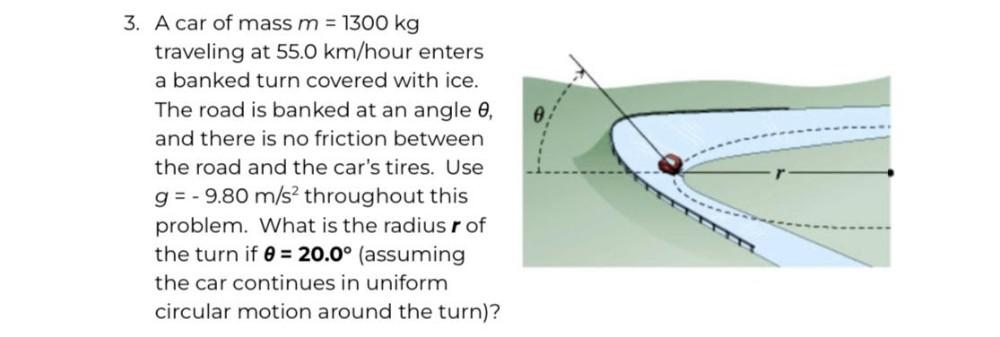Question:

# 3. A car of mass m = 1300 kg traveling at 55.0 km/hour enters a banked turn covered with ice. The road is banked at an angle 0,3. A car of mass m = 1300 kg traveling at 55.0 km/hour enters a banked turn covered with ice. The road is banked at an angle 0, and there is no friction between the road and the car's tires. Use g= -9.80 m/s2 throughout this problem. What is the radius r of the turn if 0 = 20.0° (assuming the car continues in uniform circular motion around the turn)?